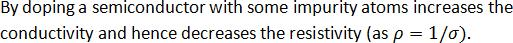Courses

# Test: Basic Electronics - 3

## 25 Questions MCQ Test Electrical Engineering SSC JE (Technical) | Test: Basic Electronics - 3

Description
This mock test of Test: Basic Electronics - 3 for Electrical Engineering (EE) helps you for every Electrical Engineering (EE) entrance exam. This contains 25 Multiple Choice Questions for Electrical Engineering (EE) Test: Basic Electronics - 3 (mcq) to study with solutions a complete question bank. The solved questions answers in this Test: Basic Electronics - 3 quiz give you a good mix of easy questions and tough questions. Electrical Engineering (EE) students definitely take this Test: Basic Electronics - 3 exercise for a better result in the exam. You can find other Test: Basic Electronics - 3 extra questions, long questions & short questions for Electrical Engineering (EE) on EduRev as well by searching above.
QUESTION: 1

Solution:
QUESTION: 2

Solution:
QUESTION: 3

### In an intrinsic semiconductor–

Solution:
QUESTION: 4

Covalent bond exists in –

Solution:
QUESTION: 5

In a good conductor, the energy gap between the conduction band and the valence band is –

Solution:
QUESTION: 6

The energy band gap of silicon is –

Solution:
QUESTION: 7

The forbidden energy band gap in conductors, semiconductor and insulators are EG1, EG2 and EG3 respectively then –

Solution:
QUESTION: 8

Which of the following is not a semiconductor?

Solution:
QUESTION: 9

Three semiconductors are arranged in the increasing order of their energy gap as follows. The correct arrangement is –

Solution:
QUESTION: 10

P-type semiconductor is prepared by doping silicon or germanium semoconductor by –

Solution:
QUESTION: 11

N-type semiconductor is obtained when silicon or germanium is doped by –

Solution:
QUESTION: 12

If a small amount of phosphorus is added to silicon–

Solution:
QUESTION: 13

P-type semiconductors are–

Solution:
QUESTION: 14

The electrical conductivity of a pure silicon or germanium sample can be increased by

Solution:
QUESTION: 15

The electrical resistivity of –

Solution:
QUESTION: 16

The addition of a very small quantity of aluminium to a silicon or germanium crystal makes it–

Solution:
QUESTION: 17

Doping of a semiconductor with small traces of impurity atoms generally changes the resistivity as follows–

Solution:QUESTION: 18

The most commonly used semiconductor is ………..

Solution:
QUESTION: 19

An intrinsic semiconductor at the absolute zero of temperature–

Solution:
QUESTION: 20

The forbidden energy gap is largest in the case of –

Solution:
QUESTION: 21

The majority carriers of electricity in a N-type semiconductor are –

Solution:
QUESTION: 22

The addition of pentavalent impurity to a semiconductor creates more–

Solution:
QUESTION: 23

Reverse bias on a junction diode–

Solution:
QUESTION: 24

The width of the depletion layer in a P-N junction diode–

Solution:
QUESTION: 25